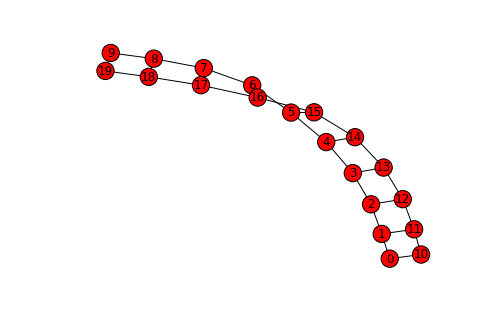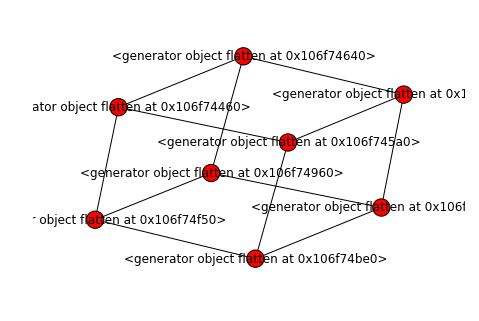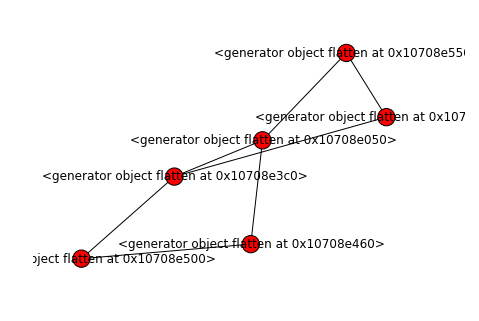In :
"""
Generators for some classic graphs.

The typical graph generator is called as follows:

>>> G=nx.complete_graph(100)

returning the complete graph on n nodes labeled 0,..,99
as a simple graph. Except for empty_graph, all the generators
in this module return a Graph class (i.e. a simple, undirected graph).

"""
#    Aric Hagberg <[email protected]>
#    Dan Schult <[email protected]>
#    Pieter Swart <[email protected]>
import itertools
__author__ ="""Aric Hagberg ([email protected])\nPieter Swart ([email protected])"""

__all__ = [ 'balanced_tree',
'barbell_graph',
'complete_graph',
'complete_bipartite_graph',
'cycle_graph',
'dorogovtsev_goltsev_mendes_graph',
'empty_graph',
'full_rary_tree',
'grid_graph',
'grid_2d_graph',
'hypercube_graph',
'lollipop_graph',
'null_graph',
'path_graph',
'star_graph',
'trivial_graph',
'wheel_graph']

#-------------------------------------------------------------------
#   Some Classic Graphs
#-------------------------------------------------------------------
import networkx as nx
from networkx.utils import is_list_of_ints, flatten

def _tree_edges(n,r):
# helper function for trees
# yields edges in rooted tree at 0 with n nodes and branching ratio r
nodes=iter(range(n))
parents=[next(nodes)] # stack of max length r
while parents:
source=parents.pop(0)
for i in range(r):
try:
target=next(nodes)
parents.append(target)
yield source,target
except StopIteration:
break

def full_rary_tree(r, n, create_using=None):
"""Creates a full r-ary tree of n vertices.

Sometimes called a k-ary, n-ary, or m-ary tree.  "... all non-leaf
vertices have exactly r children and all levels are full except
for some rightmost position of the bottom level (if a leaf at the
bottom level is missing, then so are all of the leaves to its
right." _

Parameters
----------
r : int
branching factor of the tree
n : int
Number of nodes in the tree
create_using : NetworkX graph type, optional
Use specified type to construct graph (default = networkx.Graph)

Returns
-------
G : networkx Graph
An r-ary tree with n nodes

References
----------
..  An introduction to data structures and algorithms,
James Andrew Storer,  Birkhauser Boston 2001, (page 225).
"""
G=nx.empty_graph(n,create_using)
return G

def balanced_tree(r, h, create_using=None):
"""Return the perfectly balanced r-tree of height h.

Parameters
----------
r : int
Branching factor of the tree
h : int
Height of the tree
create_using : NetworkX graph type, optional
Use specified type to construct graph (default = networkx.Graph)

Returns
-------
G : networkx Graph
A tree with n nodes

Notes
-----
This is the rooted tree where all leaves are at distance h from
the root. The root has degree r and all other internal nodes have
degree r+1.

Node labels are the integers 0 (the root) up to  number_of_nodes - 1.

Also refered to as a complete r-ary tree.
"""
# number of nodes is n=1+r+..+r^h
if r==1:
n=2
else:
n = int((1-r**(h+1))/(1-r)) # sum of geometric series r!=1
G=nx.empty_graph(n,create_using)
return G

return nx.full_rary_tree(r,n,create_using)

def barbell_graph(m1,m2,create_using=None):
"""Return the Barbell Graph: two complete graphs connected by a path.

For m1 > 1 and m2 >= 0.

Two identical complete graphs K_{m1} form the left and right bells,
and are connected by a path P_{m2}.

The 2*m1+m2  nodes are numbered
0,...,m1-1 for the left barbell,
m1,...,m1+m2-1 for the path,
and m1+m2,...,2*m1+m2-1 for the right barbell.

The 3 subgraphs are joined via the edges (m1-1,m1) and (m1+m2-1,m1+m2).
If m2=0, this is merely two complete graphs joined together.

This graph is an extremal example in David Aldous
and Jim Fill's etext on Random Walks on Graphs.

"""
if create_using is not None and create_using.is_directed():
raise nx.NetworkXError("Directed Graph not supported")
if m1<2:
raise nx.NetworkXError(\
"Invalid graph description, m1 should be >=2")
if m2<0:
raise nx.NetworkXError(\
"Invalid graph description, m2 should be >=0")

# left barbell
G=complete_graph(m1,create_using)
G.name="barbell_graph(%d,%d)"%(m1,m2)

# connecting path
if m2>1:
# right barbell
G.add_edges_from( (u,v) for u in range(m1+m2,2*m1+m2) for v in range(u+1,2*m1+m2))
# connect it up
if m2>0:
return G

def complete_graph(n,create_using=None):
""" Return the complete graph K_n with n nodes.

Node labels are the integers 0 to n-1.
"""
G=empty_graph(n,create_using)
G.name="complete_graph(%d)"%(n)
if n>1:
if G.is_directed():
edges=itertools.permutations(range(n),2)
else:
edges=itertools.combinations(range(n),2)
return G

def complete_bipartite_graph(n1,n2,create_using=None):
"""Return the complete bipartite graph K_{n1_n2}.

Composed of two partitions with n1 nodes in the first
and n2 nodes in the second. Each node in the first is
connected to each node in the second.

Node labels are the integers 0 to n1+n2-1

"""
if create_using is not None and create_using.is_directed():
raise nx.NetworkXError("Directed Graph not supported")
G=empty_graph(n1+n2,create_using)
G.name="complete_bipartite_graph(%d,%d)"%(n1,n2)
for v1 in range(n1):
for v2 in range(n2):
return G

"""Return the circular ladder graph CL_n of length n.

CL_n consists of two concentric n-cycles in which
each of the n pairs of concentric nodes are joined by an edge.

Node labels are the integers 0 to n-1

"""
return G

def cycle_graph(n,create_using=None):
"""Return the cycle graph C_n over n nodes.

C_n is the n-path with two end-nodes connected.

Node labels are the integers 0 to n-1
If create_using is a DiGraph, the direction is in increasing order.

"""
G=path_graph(n,create_using)
G.name="cycle_graph(%d)"%n
return G

def dorogovtsev_goltsev_mendes_graph(n,create_using=None):
"""Return the hierarchically constructed Dorogovtsev-Goltsev-Mendes graph.

n is the generation.
See: arXiv:/cond-mat/0112143 by Dorogovtsev, Goltsev and Mendes.

"""
if create_using is not None:
if create_using.is_directed():
raise nx.NetworkXError("Directed Graph not supported")
if create_using.is_multigraph():
raise nx.NetworkXError("Multigraph not supported")
G=empty_graph(0,create_using)
G.name="Dorogovtsev-Goltsev-Mendes Graph"
if n==0:
return G
new_node = 2         # next node to be added
for i in range(1,n+1): #iterate over number of generations.
last_generation_edges = G.edges()
number_of_edges_in_last_generation = len(last_generation_edges)
for j in range(0,number_of_edges_in_last_generation):
new_node += 1
return G

def empty_graph(n=0,create_using=None):
"""Return the empty graph with n nodes and zero edges.

Node labels are the integers 0 to n-1

For example:
>>> G=nx.empty_graph(10)
>>> G.number_of_nodes()
10
>>> G.number_of_edges()
0

The variable create_using should point to a "graph"-like object that
will be cleaned (nodes and edges will be removed) and refitted as
an empty "graph" with n nodes with integer labels. This capability
is useful for specifying the class-nature of the resulting empty
"graph" (i.e. Graph, DiGraph, MyWeirdGraphClass, etc.).

The variable create_using has two main uses:
Firstly, the variable create_using can be used to create an
empty digraph, network,etc.  For example,

>>> n=10
>>> G=nx.empty_graph(n,create_using=nx.DiGraph())

will create an empty digraph on n nodes.

Secondly, one can pass an existing graph (digraph, pseudograph,
etc.) via create_using. For example, if G is an existing graph
(resp. digraph, pseudograph, etc.), then empty_graph(n,create_using=G)
will empty G (i.e. delete all nodes and edges using G.clear() in
base) and then add n nodes and zero edges, and return the modified
graph (resp. digraph, pseudograph, etc.).

"""
if create_using is None:
# default empty graph is a simple graph
G=nx.Graph()
else:
G=create_using
G.clear()

G.name="empty_graph(%d)"%n
return G

def grid_2d_graph(m,n,periodic=False,create_using=None):
""" Return the 2d grid graph of mxn nodes,
each connected to its nearest neighbors.
Optional argument periodic=True will connect
boundary nodes via periodic boundary conditions.
"""
G=empty_graph(0,create_using)
G.name="grid_2d_graph"
rows=range(m)
columns=range(n)
G.add_nodes_from( (i,j) for i in rows for j in columns )
G.add_edges_from( ((i,j),(i-1,j)) for i in rows for j in columns if i>0 )
G.add_edges_from( ((i,j),(i,j-1)) for i in rows for j in columns if j>0 )
if G.is_directed():
G.add_edges_from( ((i,j),(i+1,j)) for i in rows for j in columns if i<m-1 )
G.add_edges_from( ((i,j),(i,j+1)) for i in rows for j in columns if j<n-1 )
if periodic:
if n>2:
G.add_edges_from( ((i,0),(i,n-1)) for i in rows )
if G.is_directed():
G.add_edges_from( ((i,n-1),(i,0)) for i in rows )
if m>2:
G.add_edges_from( ((0,j),(m-1,j)) for j in columns )
if G.is_directed():
G.add_edges_from( ((m-1,j),(0,j)) for j in columns )
G.name="periodic_grid_2d_graph(%d,%d)"%(m,n)
return G

def grid_graph(dim,periodic=False):
""" Return the n-dimensional grid graph.

The dimension is the length of the list 'dim' and the
size in each dimension is the value of the list element.

E.g. G=grid_graph(dim=[2,3]) produces a 2x3 grid graph.

If periodic=True then join grid edges with periodic boundary conditions.

"""
dlabel="%s"%dim
if dim==[]:
G=empty_graph(0)
G.name="grid_graph(%s)"%dim
return G
if not is_list_of_ints(dim):
raise nx.NetworkXError("dim is not a list of integers")
if min(dim)<=0:
raise nx.NetworkXError(\
"dim is not a list of strictly positive integers")
if periodic:
func=cycle_graph
else:
func=path_graph

dim=list(dim)
current_dim=dim.pop()
G=func(current_dim)
while len(dim)>0:
current_dim=dim.pop()
# order matters: copy before it is cleared during the creation of Gnew
Gold=G.copy()
Gnew=func(current_dim)
# explicit: create_using=None
# This is so that we get a new graph of Gnew's class.
G=nx.cartesian_product(Gnew,Gold)
# graph G is done but has labels of the form (1,(2,(3,1)))
# so relabel
H=nx.relabel_nodes(G, flatten)
H.name="grid_graph(%s)"%dlabel
return H

def hypercube_graph(n):
"""Return the n-dimensional hypercube.

Node labels are the integers 0 to 2**n - 1.

"""
dim=n*
G=grid_graph(dim)
G.name="hypercube_graph_(%d)"%n
return G

"""Return the Ladder graph of length n.

This is two rows of n nodes, with
each pair connected by a single edge.

Node labels are the integers 0 to 2*n - 1.

"""
if create_using is not None and create_using.is_directed():
raise nx.NetworkXError("Directed Graph not supported")
G=empty_graph(2*n,create_using)
return G

def lollipop_graph(m,n,create_using=None):
"""Return the Lollipop Graph; K_m connected to P_n.

This is the Barbell Graph without the right barbell.

For m>1 and n>=0, the complete graph K_m is connected to the
path P_n.  The resulting m+n nodes are labelled 0,...,m-1 for the
complete graph and m,...,m+n-1 for the path. The 2 subgraphs
are joined via the edge (m-1,m).  If n=0, this is merely a complete
graph.

Node labels are the integers 0 to number_of_nodes - 1.

(This graph is an extremal example in David Aldous and Jim
Fill's etext on Random Walks on Graphs.)

"""
if create_using is not None and create_using.is_directed():
raise nx.NetworkXError("Directed Graph not supported")
if m<2:
raise nx.NetworkXError(\
"Invalid graph description, m should be >=2")
if n<0:
raise nx.NetworkXError(\
"Invalid graph description, n should be >=0")
# the ball
G=complete_graph(m,create_using)
# the stick
if n>1:
# connect ball to stick
G.name="lollipop_graph(%d,%d)"%(m,n)
return G

def null_graph(create_using=None):
""" Return the Null graph with no nodes or edges.

See empty_graph for the use of create_using.

"""
G=empty_graph(0,create_using)
G.name="null_graph()"
return G

def path_graph(n,create_using=None):
"""Return the Path graph P_n of n nodes linearly connected by n-1 edges.

Node labels are the integers 0 to n - 1.
If create_using is a DiGraph then the edges are directed in
increasing order.

"""
G=empty_graph(n,create_using)
G.name="path_graph(%d)"%n
return G

def star_graph(n,create_using=None):
""" Return the Star graph with n+1 nodes: one center node, connected to n outer nodes.

Node labels are the integers 0 to n.

"""
G=complete_bipartite_graph(1,n,create_using)
G.name="star_graph(%d)"%n
return G

def trivial_graph(create_using=None):
""" Return the Trivial graph with one node (with integer label 0) and no edges.

"""
G=empty_graph(1,create_using)
G.name="trivial_graph()"
return G

def wheel_graph(n,create_using=None):
""" Return the wheel graph: a single hub node connected to each node of the (n-1)-node cycle graph.

Node labels are the integers 0 to n - 1.

"""
G=star_graph(n-1,create_using)
G.name="wheel_graph(%d)"%n
if n>2:
return G
In :
G=nx.complete_graph(100)
In :
%pylab inline
Populating the interactive namespace from numpy and matplotlib
WARNING: pylab import has clobbered these variables: ['flatten']
%pylab --no-import-all prevents importing * from pylab and numpy
In :
nx.draw(wheel_graph(10))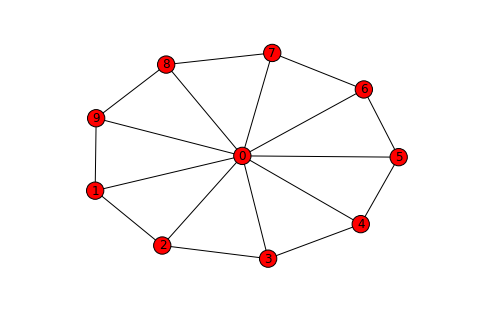In :
nx.draw(star_graph(5))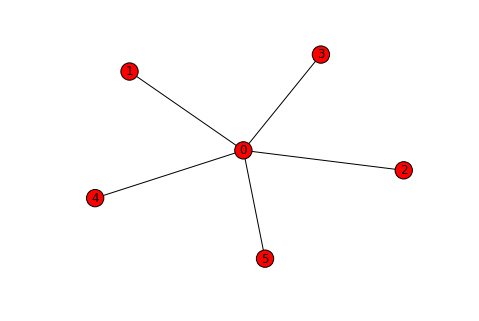In :
nx.draw(path_graph(5))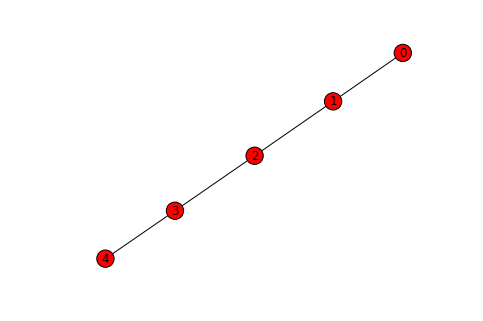In :
nx.draw(lollipop_graph(10,5))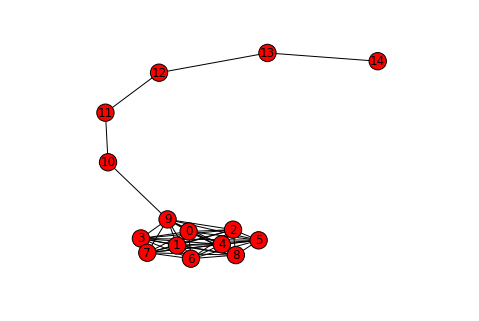In :